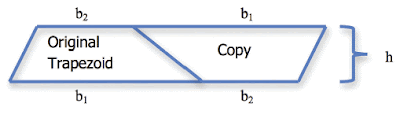## Thursday, September 1, 2011

### If You Think You Know It, Try to Teach It

Maggie Cummings—University of Utah

I am involved with a Math for America (MfA) project at the University of Utah that helps individuals with strong backgrounds in mathematics (typically a baccalaureate degree in mathematics) become secondary math teachers in high-needs schools. What has been extraordinary to me in this work is the gap between general mathematical knowledge and mathematical knowledge for teaching (MKT). This disparity has received significant attention in teacher education circles. (See, for example, Ball, Thames, and Phelps’s article, “Content Knowledge for Teaching: What Makes It Special?” in the Journal of Teacher Education 59, 2008.) The general theme of research in this area is that there is a difference between “doing” and “teaching” mathematics and that while teacher content knowledge is necessary for pedagogical knowledge and skill, the former does not guarantee the latter.

At the University of Utah, we are trying to develop a conceptual understanding of MKT at the secondary level and a means by which we might measure it. In particular, we are interested in identifying knowledge and skills that secondary mathematics teachers need but that are not necessarily possessed by those with degrees in mathematics. It may seem ridiculous to think that individuals with degrees in mathematics don’t know all the math they need for teaching secondary school students, but here are some concrete examples of where we see a gap:How can you help a seventh-grade student mentally compute 35% of 80?

Why is the area of a trapezoid ½h(b1+b2)?

Is there a geometric reason that the slopes of perpendicular lines are negative reciprocals of each other?

How might you explain why 5 minus -7 equals 12, or why the product of two negative numbers is positive?

Why is anything to the 0 power equal to 1?

When our MfA fellows begin the program, most can provide the algorithms or rules related to the above topics, but when pressed, they generally are not able to give student-friendly explanations that connect a tangible model to the algorithmic procedure. For example, fellows usually set up a proportion to solve the problem of finding 35% of 80 (x/80 = 35/100), but do not know of any way to make this problem simple enough for students to compute the answer in their head. (One approach is to understand that 35% is three and a half groups of 10% portions. Ten percent of 80 is 8, so three of them would be 24 and another half  would give 28.)

Indeed, once they examine the conceptualization, the models are not just intuitive—they actually enhance prior understandings of our fellows. The issue is that (a) these conceptualizations are vital to the work of teaching mathematics and (b) they do not seem to be developed in conjunction with typical preparation in mathematics.

It is not enough for a secondary teacher to say a negative times a negative is a positive—she must also be able to engage students in understanding why this is the case and then how this logic can be applied to other situations. In a similar vein, it is not enough that a teacher knows that a student made a mistake in simplifying an algebraic fraction; he must also be able to identify what the student was thinking in the erroneous simplification process. That way, the teacher has a better chance of helping the student connect his or her understanding of numeric fractions to algebraic fractions.

As we prepare individuals with strong backgrounds in math to become teachers, what we have learned is that advanced content knowledge in mathematics must be deliberately linked to content-specific pedagogical knowledge and skills. If that linkage is not made, advanced content knowledge stays “siloed” in the instructor, where it doesn’t do the instructor or the students much good.

Individuals wishing to teach mathematics at the secondary level need more than a strong background in advanced mathematics; they need a strong foundation in the mathematics they are going to teach. So, while it is essential that secondary math teachers understand abstract algebra, it doesn’t necessarily translate into the ability to teach basic algebra. If the truest test of understanding an idea is being able to teach it to someone else, then even some of the strongest graduating mathematics majors still have much to learn about the foundations of their chosen subject. The more they are willing to learn, the more their future students will be likely to follow suit.

About the Author: Maggie Cummings is an instructor with the Center of Science and Mathematics Education at the University of Utah. Email: margaritacummings@gmail.com

Aftermath essays are intended to be editorials and do not necessarily reflect the views of the MAA.